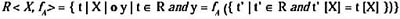# Chapter V: Operators for Multidimensional Aggregate Data

Maurizio Rafanelli, Istituto di Analisi dei Sistemi ed Informatica – C. N. R.

Italy

## OVERVIEW

In this chapter the author proposes the different approaches for defining operators able to manipulate this multidimensional structure. In particular, he initially considers operators for multidimensional aggregate data which extend relational algebra and relational calculus (the so-called enlarged relational model). Then he discusses operators for multidimensional aggregate data defined in a tabular environment. In both the cases the author defines such data as statistical (aggregate) data. Subsequently he introduces the operators for OLAP applications, giving a terminology correspondence between the multidimensional aggregate (statistical) databases and OLAP areas. Then he defines the fundamental operators deduced from the previous ones, which form the basic algebra for the manipulation of multidimensional aggregate data, giving their formal definitions and some explanatory examples.

A data model consists of a data structure, a set of operators which define an algebra, and the semantics that such operators have for this data structure. In the case of multidimensional aggregate databases, the classic relational algebra operators do not support all the possible operations needed to manipulate these complex data. Since 1982 Klug understood this limitation and proposed, in Klug (1982), an innovative operator (aggregate formation). He extended the relational algebra and the relational calculus with aggregate functions and showed that this extended language was equivalent in expressive power. He defined the aggregate formation operator in the following way. First it partitions tuples of relation R so that tuples having the same X component are in the same partition. Then the function f is applied to component A of the tuples in each partition, and the X-value and the associated aggregate value are output for each partition. Formally:

Let R D, X Atr (R), | X | = k. Let f be an aggregate function, and A, A Atr (R), be simple-valued. Thenwhere ‘o’ denotes concatenation.

Starting from this idea, other authors began to propose different models for multidimensional aggregate data (called, at that time, statistical data) and, consequently, many operators (and algebras) able to solve the problems which arose when a user tried to manipulate this kind of data. Two different approaches were followed. The first approach consisted essentially of modifying the relational algebra, and extending its operators. Among the different proposals made, those proposed in Ozsoyoglu & Ozsoyoglu (1983), in Ozsoyoglu, Ozsoyoglu, & Mata (1985), in Su (1983), in Ghosh (1986), and in Ozsoyoglu, Ozsoyoglu, & Matos (1987) are the most significant.

At the same time, other authors suggested a different approach. It consisted of formulating new operators which had an array, i.e., a multidimensional table, rather than a relation, as a reference structure. They also had the characteristics of not distinguishing between rows and columns (as a relation did) and of considering two different types of attributes with particular peculiarities: the descriptive attributes and the summary attributes. Among the proposals which refer to this second approach, we remember those presented in Rafanelli & Ricci (1984, 1985), in Fortunato, Rafanelli, Ricci, & Sebastio (1986), in Rafanelli & Shoshani (1990), and in Rafanelli & Ricci (1993). As already mentioned, these data structures can be represented in different ways: relations, istograms, graphics, cakes, tables, cubes, etc. The most widely used representation is a multidimensional table, also because it is the most common way of seeing (on a paper or on a screen) this kind of data. This manner of representing multidimensional aggregate data can often create confusion from different points of view. For example, their multidimensionality is not so evident. Different representations of the same structure (for example, exchanging rows and columns) can make the described fact seem different, while it is only the visual representation that is different—sometimes the difference between an attribute and an instance of an attribute is not clear—as explained in Rafanelli & Shoshani (1990).

For many years different algebra, and, therefore, many ad-hoc operators, were proposed, based both on a relational structure, and on a tabular structure.

In these last years, with the coinage of the new acronym OLAP (Codd, Codd, & Salley, 1993), the interest from important academic and industrial sectors has increased the amount of research in the multidimensional database area, especially from the data analysis point of view. At the heart of OLAP applications is the ability to simultaneously aggregate across many sets of dimensions. Data analysis on-line is part of this need. The request for simultaneous multidimensional aggregation was the main reason for the extension of SQL, including the "cube" operator, as proposed in Gray, Bosworth., Layman, & Pirahesh (1996) and subsequently in Gyssens & Lakshmanan (1997) and in Nguyen, Tjoa, & Wagner (2000). We will discuss the different proposals which refer both to the enlarged relational algebra and to the operators for tables, and to OLAP operators.Multidimensional Databases: Problems and Solutions
ISBN: 1591400538
EAN: 2147483647
Year: 2003
Pages: 150